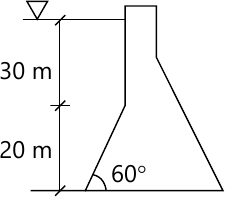GTU Civil Engineering (Semester 3)
Fluid Mechanics
December 2014
Total marks: --
Total time: --
INSTRUCTIONS
(1) Assume appropriate data and state your reasons
(2) Marks are given to the right of every question
(3) Draw neat diagrams wherever necessary

1 (a) Write statements of the following:
(i). Hydrostatic law
(ii). Pascal's law
(iii). Bernoulli's theorem
(iv). Newton's law of viscosity
(v). Archimedes principle for the buoyant force
5 M
1 (b) (i) Define the following terms:
i) Metacentric height, Kinematic viscosity, Surface tension,
Velocity potential function and Reynolds number.
5 M
1 (b) (ii) Differentiate between the following in brief:
(i) Rotational flow and Irrotational flow
(ii) Laminar flow and Turbulent flow
(iii) Compressible flow and Incompressible flow
(iv) Uniform flow and Non-uniform flow
4 M

2 (a) Derive expressions for total force and centre of pressure on a vertical plane surface submerged in static liquid.
7 M
Answer any one question from Q2 (b) & Q2 (c)
2 (b) A dam section is shown in Fig. 1. Calculate, (i) total force exerted by static water on the dam section, (ii) inclination of the force with horizontal and, (iii) depth at which this force acts. Take length of the dam section (perpendicular to the section given in Fig.1) equal to 1 m.
7 M
2 (c) Derive theoretical equation for the metacentric height of a floating body.
7 M

Answer any two question from Q3 (a), (b) & Q3 (c), (d)
3 (a) Derive Bernoulli's equation for incompressible fluid flow. State assumptions made in the derivation.
7 M
3 (b) For a fluid flow, velocity components in x and y directions are u=2xy and v=x2-y2+4 respectively. Show that the components represent a possible case of fluid flow. Derive stream function and the flow rate between the stream lines corresponding to points (1, 0) and (1, 1).
7 M
3 (c) Derive equation for rate of flow through the venturimeter.
7 M
3 (d) A sharp-edged orifice of 125 mm diameter is fixed on vertical side of a tank under a constant head of 9 m. The orifice is discharging water at a rate of 105 liters/sec. A point on the jet has horizontal and vertical coordinates of 4.25 m and 0.55 m respectively, which are measured from the vena contracta. Calculate coefficient of velocity, coefficient of discharge and coefficient of contraction. Also estimate area of the jet at the vena contracta.
7 M

Answer any two question from Q4 (a), (b) & Q4 (c), (b)
4 (a) Derive equation for discharge over a rectangular weir. Also explain significance of velocity of approach.
7 M
4 (b) (i) Calculate capillary effect in a glass tube of 3 mm diameter when immersed in (i) water and, (ii) mercury (specific gravity = 13.6) at temperature of 20°C. The surface tension of water and mercury at temperature of 20°C are 0.074 N/m and 0.52 N/m. The contact angles water and mercury are 0° and 130° respectively. Take specific weight of water at 20°C as equal to 9.8 KN/m3.
4 M
4 (b) (ii) Define compressibility of a fluid. When the pressure of liquid is increased to 7.5×103 KN/m2 from 4×103 KN/m2, its volume is found to reduce by 0.075 percent. Calculate the bulk modulus of elasticity of the liquid.
3 M
4 (c) Differentiate between a stream lined body and a bluff body. Prove that the coefficient of drag for the drag on sphere is given by CD=24/Re, when Re (Reynold's number) ≤ 0.2.
7 M
4 (d) Experiments on a flat plate of 1 m length and 0.5 m width were conducted in a wind tunnel in which wind was blowing horizontally at a speed of 60 Km/hour. The plate was kept at such an angle that the coefficients of drag and lift were 0.2 and 0.88 respectively. Calculate, (i) drag and lift forces, (ii) resultant force and its direction and, (iii) power exerted by the air stream on the plate. Take specific weight of air equal to 11.28 KN/m3.
7 M

Answer any two question from Q5(a), (b) & Q5 (c), (d)
5 (a) Derive equation for sonic velocity of sound wave in a compressible fluid in terms of the bulk modulus of elasticity of the fluid medium.
7 M
5 (b) An aeroplane is flying at 950 Km/hour through still air having an absolute pressure of 80 KN/m2 and temperature -7°C. Calculate stagnation pressure, stagnation temperature and stagnation density, on the stagnation point on the nose of the plane. Take R=287 J/ Kg K and γ=1.4 for air.
7 M
5 (c) Derive continuity equation for three dimensional incompressible flow.
7 M
5 (d) (i) An inverted differential manometer, having an oil of specific gravity 0.8 as manometric liquid, is connected two pipes A and B which are at same level and both carrying water. Level of the oil in left limb is 0.2 m above centre of pipe A and, level of the oil in right limb is 0.45 m above centre of pipe B. Calculate difference in pressure between the two pipes.
4 M
5 (d) (ii) A solid cylinder having 1.5 m diameter and 2 m height is floating in water with its axis vertical. If the specific gravity of material of cylinder is 0.85, calculate metacentric height and state whether the equilibrium is stable or unstable.3 M

More question papers from Fluid Mechanics